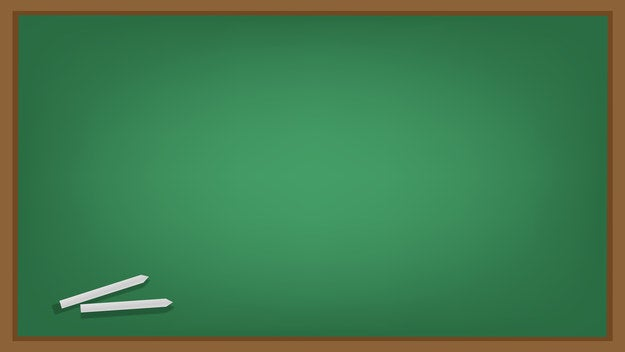1. What makes a polygon?Getty Images
Correct!
Wrong!

A polygon is a plane figure with at least three straight sides and angles, and usually five or more.Via Getty Images
2. Which one describes a "vertex"?Getty Images
Correct!
Wrong!

A vertex is the intersection point of two sides of plain figure.Via BuzzFeed
3. Which one is a scalene triangle?Getty Images
Correct!
Wrong!

A scalene triangle is a triangle that has three unequal sides.Via Getty Images
4. Which of these do you multiply by pi to get the area of a circle?Getty Images
Correct!
Wrong!

To find the area of a circle, you must multiply pi by the radius squared.

5. What kind of angle is less than 90 degrees?Getty Images
Correct!
Wrong!

An acute angle is less than 90 degrees.Via Getty Images
6. The Pythagorean Theorem is used to determine the length of which shape's side?Getty Images
Correct!
Wrong!

If you know the length of two sides of a right triangle, the Pythagorean Theorem will determine the length of the third side.Via BuzzFeed
7. Which describes the part of a straight line that it originates and extends from?Getty Images
Correct!
Wrong!

A ray is the originating point of a line it extends from.Via BuzzFeed
8. How do you find the cosine of an angle on a right triangle?Getty Images
Correct!
Wrong!

To find the cosine of an angle, you must divide the adjacent side by the hypotenuse.

9. Which describes all points that are on the same line?Getty Images
Correct!
Wrong!

Collinear points are three or more points that lie on a straight line.Via BuzzFeed
10. Which describes "congruent"?Getty Images
Correct!
Wrong!

Congruent means figures that have the same measurements for their angles and sides.Via BuzzFeed